• 常见幂级数求和函数方法： 先导后积(n在分母：∑2+∞xnn(n−1))，先积后导(n在分子：∑0+∞nxn−1)，微分方程法(n在分子：∑0+∞(n+1)xnn!)先导后积(n在分母：\sum_{2}^{+\infty} \frac{x^n}{n(n-1)})，\\ 先积后导...
幂级数公式
常见幂级数求和函数方法：
$先导后积(n在分母：\sum_{2}^{+\infty} \frac{x^n}{n(n-1)})，\\ 先积后导(n在分子：\sum_{0}^{+\infty} nx^{n-1})，\\ 微分方程法(\sum_{0}^{+\infty} \frac{(n+1)x^n}{n!})\\ 柯西乘法公式("多项式乘法")：\sum a_nx^n,\sum b_nx^n绝对收敛\\则\sum a_nx^n*\sum b_nx^n=\sum c_nx^n绝对收敛\$/extract_itex] 微分方程法 幂级数的和函数微分后，再与原来幂级数作某种运算，得到一个含有幂级数和函数以及 和函数导数的关系式，即微分方程。最后求解此微分方程即得和函数。 微分方程法例1: $S(x)=\sum_0^{+\infty}\frac{n+1}{n!}x^n\\ 由题意得S(x)-S'(x)=(1+x+\frac{x^2}{2}+\frac{x^3}{3!}+\frac{x^4}{4!}+……)\\ S(x)=e^x(x+1)$ 微分方程法例2:级数系数间的递推关系与微分方程 $a_1=1,a_{n+1}=f(a_n),求S(x)\\ 则S'(x)=(a_1x+\sum_{n=2}^{+\infty} a_nx^n)'\\ =a_1+\sum_{n=2}^{+\infty} a_n* n *x^{n-1}\\ =a_1+\sum_{n=1}^{+\infty} a_{n+1}*( n+1) *x^{n}\\ =a_1+\sum_{n=1}^{+\infty} f( a_{n})*( n+1) *x^{n}\\ f( a_{n})*( n+1) 如果能写成 a_n +na_n+g(n)的形式(其中g(n)x^n为某函数的幂级数展开\\ 则=a_1+\sum_{n=1}^{+\infty} (a_n +na_n+g(n) )*x^{n}\\ =a_1+\sum_{n=1}^{+\infty} (a_n )*x^{n}+\sum_{n=1}^{+\infty} (na_n)*x^{n}+\sum_{n=1}^{+\infty} (g(n) )*x^{n}\\ =a_1+S(x)+xS'(x)+\sum_{n=1}^{+\infty} (g(n) )*x^{n}\\$ 柯西乘法公式： $S(x)=\sum_{1}^n- (1+\frac{1}{2}+\frac{1}{3}+\frac{1}{4}+……+\frac{1}{n}) x^n\\ 观察x^n的系数c_n，-(1),-(1+\frac{1}{2}),-(1+\frac{1}{2}+\frac{1}{3})……\\ ln(1-x)=-(x+\frac{x^2}{2}+\frac{x^3}{3}+\frac{x^4}{4}+……+\frac{x^n}{n})\\ \frac{1}{1-x}=1+x+x^2+……+x^n\\ S(x)=\frac{ln(1-x)}{1-x}$  展开全文• 文章目录生成函数引言定义有关幂级数的有用事实形式幂级数幂级数的和、积广义二项式定理常见的生成函数使用生成函数解决计数问题使用生成函数求解递推关系使用生成函数证明恒等式 生成函数 引言 定义 实数序列a0、a1... 文章目录生成函数引言定义有关幂级数的有用事实形式幂级数幂级数的和、积广义二项式定理常见的生成函数使用生成函数解决计数问题不定方程的解的个数完全背包问题的方案数有顺序的背包问题的方案数使用生成函数求解递推关系使用生成函数证明恒等式（略） 生成函数 引言 定义 实数序列$a_0、a_1、a_2、……、a_n$的生成函数,是无穷级数 $G(x)=\sum_{k=0}^{\infty} a_k*x^{k}=a_0+a_1*x+a_2*x^2+……+a_k*x^k+……$ 上述的生成函数也被称为普通生成函数，因为还有其他类型的生成函数。 也就是说，我们将一个数的序列对应到一个函数，而这个函数的幂级数展开的各项系数就是这个序列。 有关幂级数的有用事实 形式幂级数 如果将级数的收敛问题忽略掉，也就是不关心幂级数的收敛域，这样的级数就称为形式幂级数。 幂级数的和、积 若$f(x)=\sum_{k=0}^{\infty} a_k*x^{k}$,$g(x)=\sum_{k=0}^{\infty} b_k*x^{k}$； 则， 和 $f(x)+g(x)=\sum_{k=0}^{\infty} (a_k+b_k)*x^{k}$ 积 $f(x)g(x)=\sum_{k=0}^{\infty} (\sum_{j=0}^k a_j*b_{k-j})*x^{k}$ 广义二项式定理 广义二项式系数 设$u$是实数，且$k$是非负整数。那么广义二项式系数 $\binom{u}{k}= \begin{cases} u(u-1)*……*(u-k+1), k>0\\ 1,k= 0\\ \end{cases}$ 当u为负整数，广义二项式系数为 $\binom{-n}{r}=C(-n,r)=(-1)^rC(n+r-1,r)$ 广义二项式定理 $(1+x)^u=\sum_{k=0}^{\infty}\binom{u}{k}x^k$ ps 当u是正整数是，就是普通的二项式定理，因为$C(u,k),u>k$时为0。 常见的生成函数 微积分中经典的级数 G(x) $a_k$ $ln(1+x) =x-x^2/x+x^3/3$+…… $(-1)^{k+1}/k$ $e^x=\sum_{k=0}^{\infty}x^k/k!=1+x+x^2/2+x^3/3!+……$ $1/k!$ 二项式定理相关 G(x) $a_k$ $(1+x)^n=\sum_{k=0}^{\infty}C(n,k)x^k$ $C(n,k)$ $(1+ax)^n=\sum_{k=0}^{\infty}a^kC(n,k)x^k$ $C(n,k)a^k$ 广义二项式定理相关 G(x) $a_k$ $1/(1-x)=\sum_{k=0}^{\infty}x^k$ 1 $1/(1-ax)=\sum_{k=0}^{\infty}a^kx^k$ $a^k$ $1/(1-x^r)=\sum_{k=0}^{\infty}x^{rk}$ $1/(1-x)^2=\sum_{k=0}^{\infty}(k+1)x^k$ $k+1$ $1/(1-x)^n=\sum_{k=0}^{\infty}C(n+k-1,k)x^k$ $C(n+k-1,k)$ 使用生成函数解决计数问题 不定方程的解的个数 $e_1+e_2+……+e_n=m$ 求非负整数解的方案数。 可以用隔板法求出得$C(m+n-1,m)$。 利用生成函数的思想： $(1+x^1+x^2+……x^m)(1+x^1+x^2+……+x^m)(1+x^1+x^2+……+x^m)(1+x^1+x^2+……+x^m)……（n项）$ 那么$x^m$项的系数就是原问题的方案数。 这在$e_1、e_2、……$有约束限制的时候，更显优势。 比如 $e_1+e_2+……+e_3=17$，且$2<=e1<=5、3<=e2<=6、4<=e3<=7$ 那么上述解的个数是 $(x^2+x^3+x^4+x^5)(x^3+x^4+x^5+x^6)(x^4+x^5+x^6+x^7)$的$x^{17}$项的系数。 有这样的一个例子： 有一堆的1元、2元、5元硬币，数量不限，用它们组和成总和为$r$元。 有多少方案。 完全背包问题的方案数 动态规划解法 这其实是一个完全背包问题求方案数的问题。 $f[i][j] = \sum_{k=0}^{maxK} f[i-1][j-k*v]$ 当然可以再使用递推关系式优化成 $f[i][j]$ = $f[i-1][j]+f[i][j-v]$ 生成函数解法 仿照我们上面的解法， 方案数就是 $(1+x+x^2+x^3+……)(1+x^2+x^4+x^6+……)(1+x^5+x^{10}+x^{15}+……)$ 的$x^r$的系数。 第一项表示选择1元硬币，比如第一项中的$x^7$表示选了7个一元硬币，如此推知，每一项实际上对应着DP过程中考虑每一个物品。 有顺序的背包问题的方案数 动态规划解法 这种解法类似于跳楼梯问题，每次跳一级或者两级，跳到100级有多少中跳法。 递推方程：$f[i]=f[i-1]+f[i-2]+f[i-5]$ 生成函数解法 考虑恰好一共选了$n$个硬币产生$r$元的方案数，因为考虑了顺序， 所以此时的方案数为$(x+x^2+x^5)^n$中$x^r$的系数 因为可以用任意多次硬币去形成$r$元，所以按照加法原理。 总方案数是 $1+(x+x^2+x^5)+(x+x^2+x^5)^2+(x+x^2+x^5)^3+……$的$x^r$的系数。 使用生成函数求解递推关系 通过寻找生成函数的显式公式来求解满足递推关系和初始条件的解。 不过这种方案具有很大的局限性。 如果可以使用求解线性递推关系的那种方案，生成函数法并不是首选方案。 举一例说明： $a_n=8*a_{n-1}+10^{n-1}①$ 两边同乘$x^n$: $a_n*x^n=8*a_{n-1}x^n+10^{n-1}②$ 两边从$n=1$开始求和: $G(x)-1 = \sum_{n=1}^{\infty}a_n*x_n\\ =\sum_{n=1}^{\infty}(8*a_{n-1}*x^n+10^{n-1}*x^n)\\ =8x\sum_{n=1}^{\infty}(a_{n-1}*x^{n-1})+x*\sum_{n=1}^{\infty}10^{n-1}x^{n-1}\\ =8xG(x)+x/(1-10x)$ 求出 $G(x)=(1-9x)/((1-8x)(1-10x))\\ = 1/2(1/(1-8x)+1/(1-10x))\\ = 1/2(\sum_{0}^{\infty}8^n+\sum_{0}^{\infty}10^{n})x^n\\$ 所以， $a_n=1/2(8^n+10^n)$ 使用生成函数证明恒等式（略）  展开全文• 其次,通过探讨常见的离散型随机变量分布在幂级数分布族下的参数结构,研究表明常见的离散型随机变量的分布均属于幂级数分布族;最后应用幂级数分布族性质确定这些离散型分布的完备充分统计量的分布形式、参数的一致... • 级数收敛：如果级数∑i=1∞ui\sum_{i=1}^\infty u_i∑i=1∞​ui​的部分和数列{sn}\{s_n\}{sn​}有极限s，即 limn→∞sn=s lim_{n\to \infty}s_n=s limn→∞​sn​=s 那么称无穷级数∑i=1∞ui\sum_{i=1}^\infty u_i... 级数收敛：如果级数$\sum_{i=1}^\infty u_i$的部分和数列$\{s_n\}$有极限s，即 $lim_{n\to \infty}s_n=s$ 那么称无穷级数$\sum_{i=1}^\infty u_i$收敛，这时极限是叫做这级数的和，并写出 $s=u_1+u_2+···+u_i+···；$ 如果${s_n}$没有极限，那么称无穷级数$\sum_{i=1}^\infty u_i$发散。 1、函数项级数的概念 如果给定一个定义在区间I上的函数列 $u_1(x),u_2(x),u_3(x),···,u_n(x),···$ 那么由这函数列构成的表达式 $u_1(x)+u_2(x)+u_3(x)+···+u_n(x)+··· \tag{1}$ 称为定义在区间I上的（函数项）无穷级数，简称（函数项）级数。 对于每一个确定的值$x_0\in I$，函数项级数（1）成为常数项级数 $u_1(x_0)+u_2(x_0)+u_3(x_0)+···+u_n(x_0)+··· \tag{2}$ 这个级数（2）可能收敛也可能发散。如果级数（2）收敛，就称点$x_0$是函数项级数（1）的收敛点；如果级数（2）发散，就称点$x_0$是函数项级数（1）的发散点。函数项级数（1）的收敛点的全体称为它的收敛域，发散点的全体称为它的发散域。 对应于收敛域内的任意一个数x，函数项级数称为一收敛的常数项级数，因而有一确定的和s。这样，在收敛域上，函数项级数的和是x的函数$s(x)$，通常称$s(x)$为函数项级数的和函数，这函数的定义域就是级数的收敛域，并写成 $s(x)=u_1(x)+u_2(x)+u_3(x)+···+u_n(x)+···$ 把函数项级数（1）的前n项的部分和记作$s_n(x)$，则在收敛域上有 $lim_{n\to \infty}s_n(x)=s(x)$ 记$r_n(x)=s(x)-s_n(x)$，$r_n(x)$叫做函数项级数的余项（当然，只有x在收敛域上$r_n(x)$才有意义），并有 $lim_{n\to \infty}r_n(x)=0$ 2、幂级数的定义 函数项级数中简单而常见的一类级数就是各项都是常数乘幂函数的函数项级数，即所谓幂级数，它的形式是 $\sum_{n=0}^\infty a_nx^n=a_0+a_1x+a_2x^2+···+a_nx^n+···，$ 其中常数$a_0,a_1,a_2,···，a_n,···$叫做幂级数的系数。 性质1：幂级数$\sum_{n=0}^\infty a_nx^n$的和函数$s(x)$在其收敛域I上连续。 性质2：幂级数$\sum_{n=0}^\infty a_nx^n$的和函数$s(x)$在其收敛域I上可积，并有逐项积分公式 $\int_0^xs(t)dt=\int_0^x[\sum_{n=0}^\infty a_nt^n]dt=\sum_{n=0}^\infty\int_0^xa_nt^ndt =\sum_{n=0}^\infty\frac{a_n}{n+1}x^{n+1}(x\in I)$ 逐项积分后所得到的幂级数和原级数有相同的收敛半径。 性质3：幂级数$\sum_{n=0}^\infty a_nx^n$和和函数$s(x)$在其收敛区间$(-R,R)$内可导，且有逐项求导公式 $s'(x)=(\sum_{n=0}^\infty a_nx^n)’=\sum_{n=0}^\infty(a_nx^n)'=\sum_{n=1}^x na_nx^{n-1}(|x| 逐项求导后所得到的幂级数和原级数有相同的收敛半径。 结论：幂级数$\sum_{n=0}^\infty a_nx^n$的和函数$s(x)$在其收敛区间$(-R,R)$内具有任意阶导数 3、函数展开成幂级数 给定函数$f(x)$，要考虑它是否能在某个区间“展开成幂级数”，就是说，是否能找到这样一个幂级数，它在某区间收敛，且其和恰好就是给定的函数$f(x)$。如果能找到这样的幂级数，则函数$f(x)$在该区间能展开成幂级数，而这个幂级数在该区间就表达了函数$f(x)$。 假设函数$f(x)$在点$x_0$的某邻域$U(x_0)$内能展开成幂级数，既有 $f(x)=a_0+a_1(x-x_0)+a_2(x-x_0)^2+···+a_n(x-x_0)^n+···,x\in U(x_0) \tag{1}$ 则根据和函数的性质，可知$f(x)$在$U(x_0)$内应具有任意阶导数，且 $f^{(n)}(x)=n!a_n+(n+1)!a_{n+1}(x-x_0)+\frac{(n+2)!}{2!}a_{n+2}(x-x_0)^2+···，$ 由此可得 $f^{n}(x_0)=n!a_n$ 于是 $a_n=\frac{1}{n!}f^{(n)}(x_0) \quad (n=0,1,2,···) \tag{2}$ 这就表明，如果函数$f(x)$有幂级数展开式（1），那么该幂级数的系数$a_n$由公式（2）确定，即该幂级数必为 $f(x_0)+f'(x_0)(x-x_0)+···+\frac{1}{n!}f^{(n)}(x_0)(x-x_0)^n+··· \\ =\sum_{n=0}^\infty \frac{1}{n!}f^{(n)}(x_0)(x-x_0)^n \tag{3}$ 而展开式必为 $\sum_{n=0}^\infty\frac{1}{n!}f^{(n)}(x_0)(x-x_0)^n,x\in U(x_0) \tag{4}$ 幂级数（3）叫做函数$f(x)$在点$x_0$处的泰勒级数，展开式$(4)$叫做函数$f(x)$在点$x_0$处的泰勒展开式。 由以上讨论知，函数$f(x)$在$U(x_0)$内能展开成幂级数的充分必要条件是泰勒展开式（4）成立，也就是泰勒级数（3）在$U(x_0)$内收敛，且收敛到$f(x)$。 定理：设函数$f(x)$在点$x_0$的某一邻域$U(x_0)$内具有各阶导数，则$f(x)$在该邻域内能展开成泰勒级数的充分必要条件是在该邻域内$f(x)$的泰勒公式中的余项$R_n(x)$当$n\to \infty$时的极限为零，即 $lim_{n\to \infty}R_n(x)=0, \quad x\in U(x_0)$ 在（3）式中，取$x_0=0$，得 $f(0)+f'(0)x+···+\frac{1}{n!}f^{(n)}(0)x^n+···=\sum_{n=0}^\infty\frac{1}{n!}f^{(n)}(0)x^n \tag{5}$ 级数（5）称为函数$f(x)$的麦克劳林级数。若$f(x)$能在$(-r,r)$内展开成x的幂级数，则有 $f(x)=\sum_{n=0}^\infty\frac{1}{n!}f^{(n)}(0)x^n \quad (|x| （6）式称为函数$f(x)$的麦克劳林展开式。  展开全文• 这是一种特殊的矩阵级数，之前矩阵级数中，计算的是下面这种AkA_kAk​，而在方阵幂级数中，计算的是AkA^kAk 对比之前学到的幂级数，ZkZ^kZk和AkA^kAk有什么关系呢？ 假设 证明 解决了幂级数的收敛性判定问题：... 定义 这是一种特殊的矩阵级数，之前矩阵级数中，计算的是下面这种$A_k$，而在方阵幂级数中，计算的是$A^k$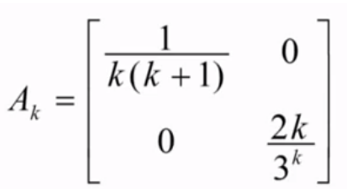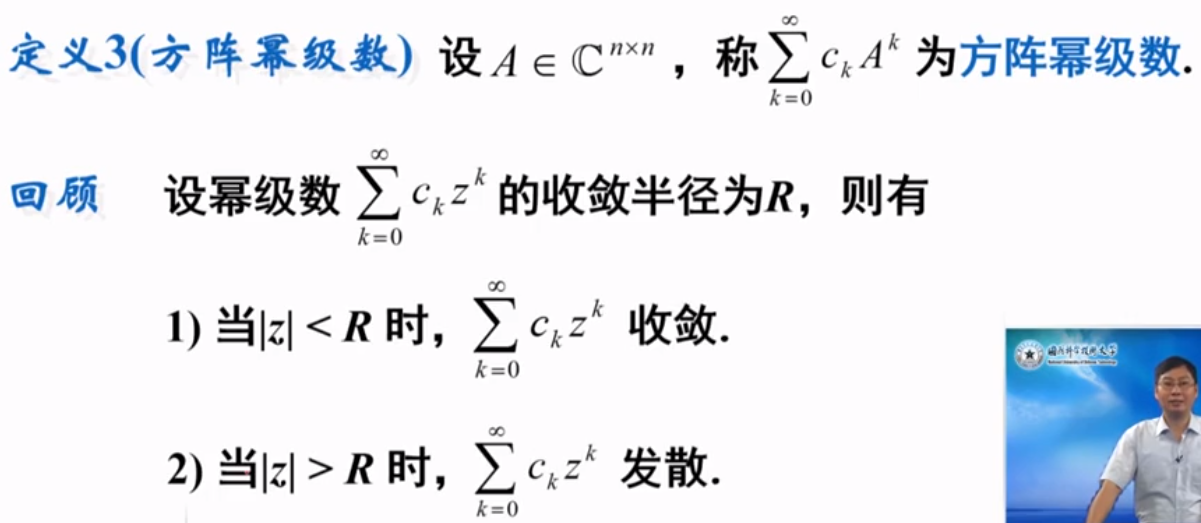对比之前学到的幂级数，$Z^k$和$A^k$有什么关系呢？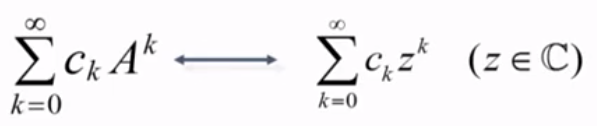假设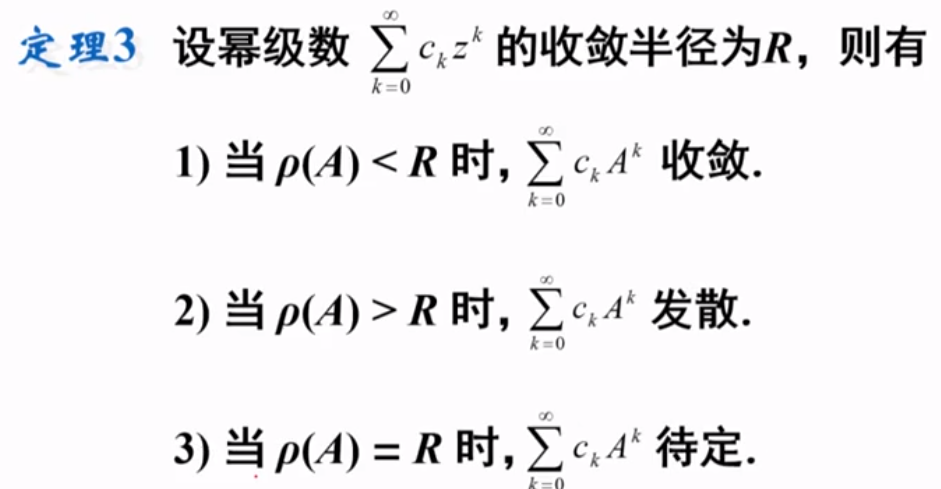证明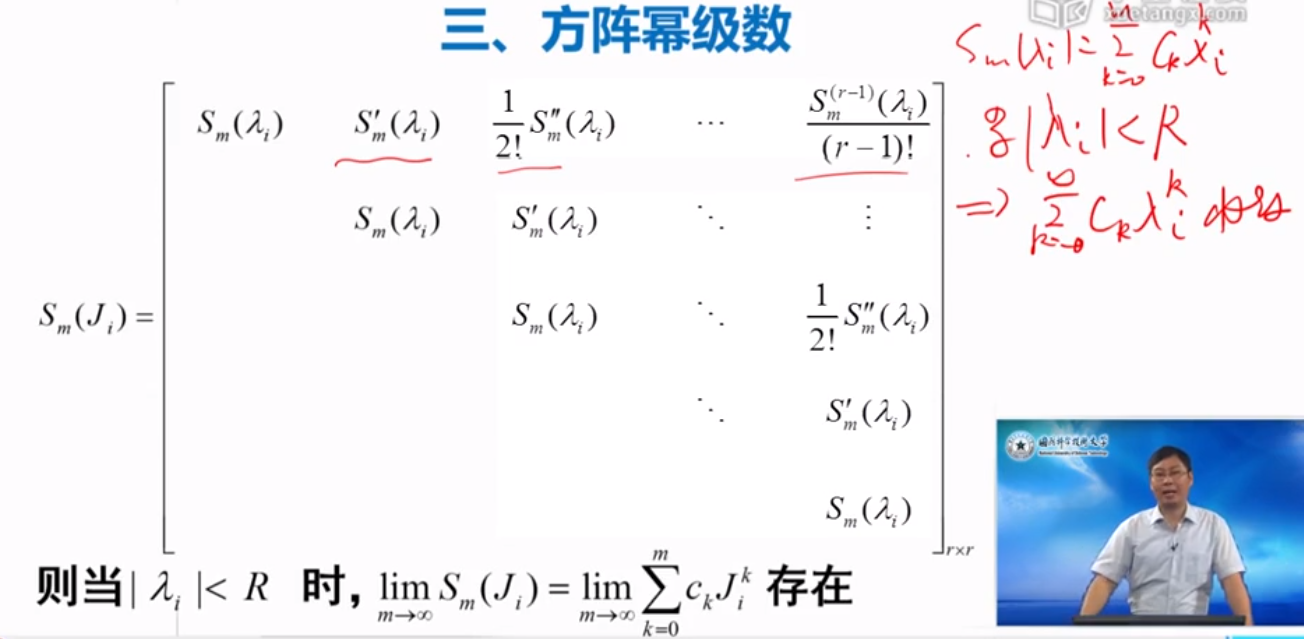解决了幂级数的收敛性判定问题：谱半径和函数收敛半径的关系。 例子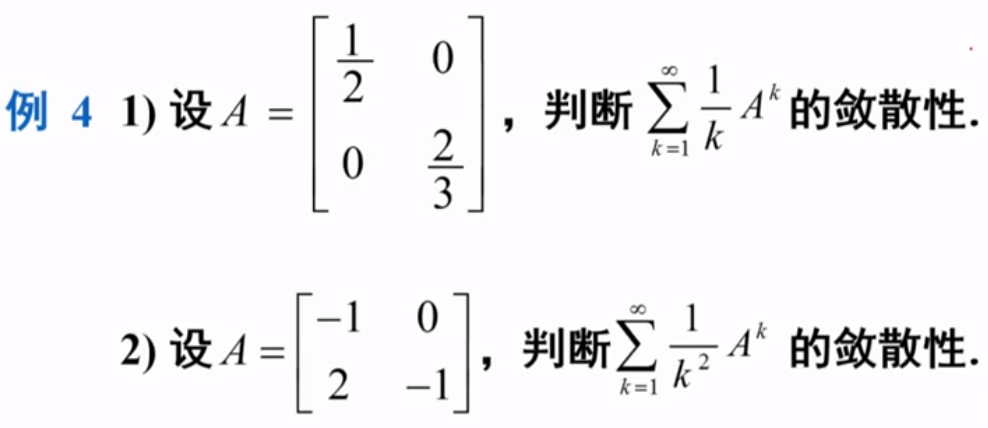因为相等了，所以要实际计算。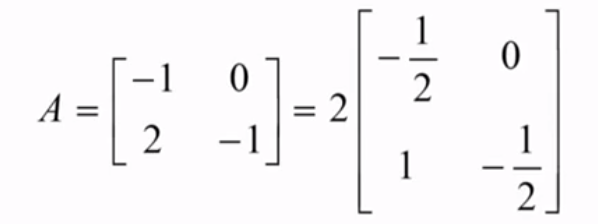把$A$变成Jordan标准型，就好算了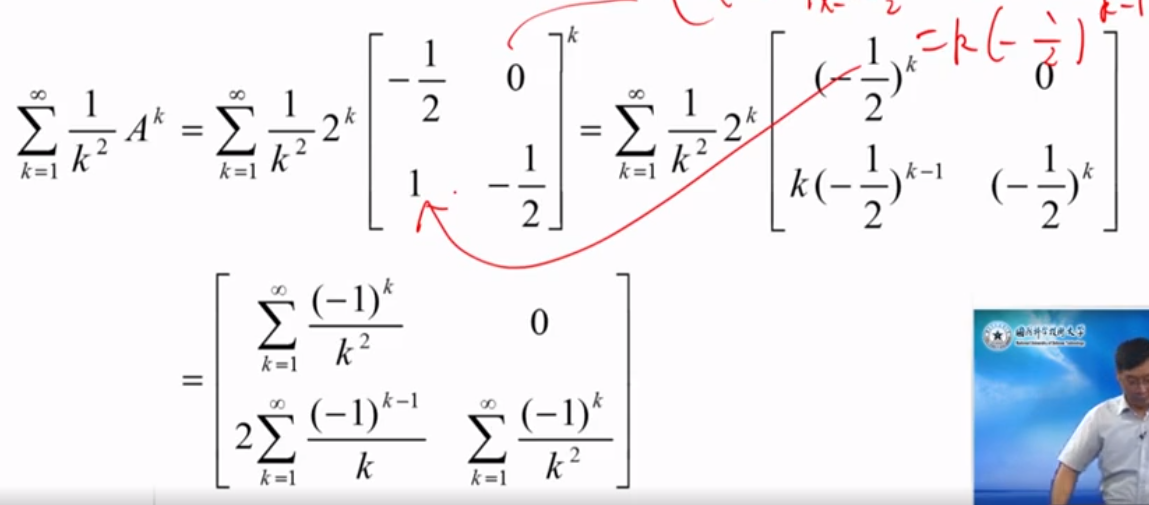细节：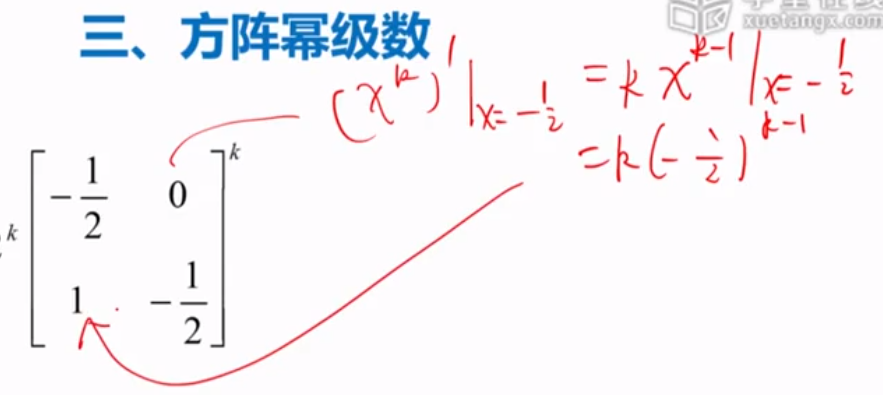第二行第一列如何没有上面的$(-1)^k$，这就是调和基数，是发散的。有$(-1)^k$就变成了交错级数，用莱布尼兹判别法，这个就是收敛的。 交错级数敛散性判别  展开全文矩阵论 • 利用级数求和推导泊松分布的期望方差@(概率论)闲来无事，动手推导一个常见的泊松分布的表达式。回顾泊松分布：设变量X服从λ\lambda的泊松分布，则：P(X=k)=λkk!e−λ,k=0,1,2,....P(X = k) = \frac{\lambda^k}{k!}...泊松分布推导 • 幂级数难题整理 1、求已知函数的幂级数展开式 ex1−x\frac{{e}^{x}}{1-x}1−xex​ 解：由于 ex=∑n=0∞xnn!,x∈(−∞,+∞) 及 11−x=∑n=0∞xn,x∈(−1,1) 所以 ex1−x=(∑n=0∞xnn!)(∑n=... • 级数通常指无穷级数，而有穷级数通常称为数列。数列/级数求和或求近似值是常见的循环编程练习。 下面将给出一些题目。 • 现给定一个实数x，要求利用此幂级数部分和求ex的近似值，求和一直继续到最后一项的绝对值小于0.00001。 输入格式: 输入在一行中给出一个实数x∈[0,5]。 输出格式: 在一行中输出满足条件的幂级数部分和，保留小数点后...c语言 • 常见级数形式，如下所示。 S ：级数的和 i： 自变量，值域为[a,b] f(i)：为关于自变量i的函数 函数： symsum 功能： 级数符号求和 语法： symsum(S) symsum(S,V) symsum(S,a,b) ... • 现给定一个实数x，要求利用此幂级数部分和求ex的近似值，求和一直继续到最后一项的绝对值小于0.00001。 输入格式: 输入在一行中给出一个实数x∈[0,5]。 输出格式: 在一行中输出满足条件的幂级数部分和，保留小数点后...c语言 • ## 常见函数的级数展开及推导 千次阅读 多人点赞 2020-09-19 19:39:18 • 幂级数求和有很多方法，如常见的逐项求导逐项积分，或者其他的方法 这里想一种情况，将级数求和问题转换成微分方程，通过解出微分方程的通解，间接的求出了级数的和。 思路是这样的，通过拆分级数的形式，加加减减...微分方程 考研数学 • 幂级数（幂函数级数） 幂级数的收敛域和收敛半径 和函数的求法与展开 微积分法求和函数 和函数的展开 马克劳林展开 必须记住的展开 1. 正项级数和交错级数 1.1 级数的定义和性质 级数的定义 什么是级数，就是... • 一、十一个组合恒等式 、 二、组合恒等式 证明方法 、 三、组合数 求和 ∑ 方法组合恒等式 • 0. 前置1. 广义二项式定理(牛顿二项式定理)我们重新定义...$其中 $$r^{\underline{k}}$$ 是下降，$$r\in\mathbb C,k\in\mathbb N$$ .注意 $$r\in\mathbb C$$ . 在这种情况下，对于任意 $$\alpha\in\mathbb C$$...
• 后来经过推广，我们得到了自然数求和的通式： 我们既然找到了自然数的求和公式，就不妨再去推广一下，试着寻找一下推广的求和公式： 利用二项式定理计算 的封闭式为了算这个式子，我们不妨先研究一下这个求和式： ...
• 1、复杂度分析的主要方法 1)迭代:技术求和 2)递归: 递归跟踪+递推方程 3)猜测+验证 ...2)级数 : 比次高出一阶 T2(n) = 12 + 22 + 32 + ……+n2 = n(n+1)(2n+1)/6 = Ο(n3) T3(n) ...
• Matlab 软件的应用 前言 Newton 和 Leibnitz 创立的微积分学是很多科学科学的基础本课程将借助 MATLAB 语言的符号运算工具箱可以直接对微积分学中最常见的问题如单变量与多变量微积分极限级数求和Taylor幂级数展开...
• ## 泰勒级数的理解

千次阅读 2018-02-13 10:56:36
展开前求解函数的值很困难，展开后是函数的线性组合，虽然有很多很多项，但是每一项都是函数，因此每一项都容易求解。于是只要对展开后的求和，就能得到展开前的函数的值。机器学习算法的本质上是优化问题求解，...
• 发散级数(中文维基百科) 发散级数 （英语：Divergent Series）指（按柯西意义下）不收敛的级数。如级数 1+2+3+4+⋯1 + 2 + 3 + 4 + \cdots1+2+3+4+⋯和 1−1+1−1+⋯{\displaystyle 1-1+1-1+\cdots }1−1+1−1+⋯ ，...
• 考研数学各科的“命题潜规则”，看完多得好几分！...如幂级数求和展开，记住常见的几个泰勒级数公式，会通过基本变形或求导求积把已知函数（或级数）朝常见公式转化，这类问题就基本解决了。而线代不机器学习 人工智能 python 数据分析
• 幂级数收敛 幂级数收敛求收敛半径一般是lim⁡n→∞an+1an=p,r=1p\lim\limits_{n\to\infty}\frac{a_{n+1}}{a_{n}}=p,r=\frac{1}{p}n→∞lim​an​an+1​​=p,r=p1​或者开n次以后求极限。 但是对于抽象幂级数，可能先...
• 数学分析基本功 一、求和 1.1 总论 ​ 求和的地位跟极限类似，是数学分析中的基础操作，因为利用求和，可以沟通柯西收敛原理，又是级数的预处理步骤，所以总结求和的方法就显得...​ 这个没有办法用数项级数求和的办法
• 序列另一个常见的应用是对其进行求和，这在算法分析上尤为常见，基本的算术级数和几何级数都有求和公式，平方和立方和等公式也要博闻强记，熟练掌握。求和运算中一个常用的技巧是下标替换。数学 离散数学
• 文章目录第十章 无穷级数10.1 数项级数10.2 数项级数敛散性10.3 函数项级数10.4 傅里叶级数 第十章 无穷级数 10.1 数项级数 柯西收敛定理（序列极限的充要条件）：∀ϵ>0,∃N,s.t.∣an−am∣<ϵ,只要n≥N,m...
• 背景： OZY\text{OZY}OZY师兄来diss\text{diss}diss我们了。 正题： ...级数： 级数是指将一个无穷数列 的项依次用加号连接起来的函数。...当n→∞,fn→0n→\infty,f_n→0n→∞,fn...幂级数： 形如∑n=0∞an(x−x0)...生成函数...